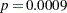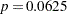Previous Page | Next Page

 The GLIMMIX Procedure

## Example 38.7 Isotonic Contrasts for Ordered Alternatives

Dose response studies often focus on testing for monotone increasing or decreasing behavior in the mean values of the dependent variable. Hirotsu and Srivastava (2000) demonstrate one approach by using data that originally appeared in Moriguchi (1976). The data, which follow, consist of ferrite cores subjected to four increasing temperatures. The response variable is the magnetic force of each core.

```data FerriteCores;
do Temp = 1 to 4;
do rep = 1 to 5; drop rep;
input MagneticForce @@;
output;
end;
end;
datalines;
10.8  9.9 10.7 10.4  9.7
10.7 10.6 11.0 10.8 10.9
11.9 11.2 11.0 11.1 11.3
11.4 10.7 10.9 11.3 11.7
;
```

It is of interest to test whether the magnetic force of the cores rises monotonically with temperature. The approach of Hirotsu and Srivastava (2000) depends on the lower confidence limits of the isotonic contrasts of the force means at each temperature, adjusted for multiplicity. The corresponding isotonic contrast compares the average of a particular group and the preceding groups with the average of the succeeding groups. You can compute adjusted confidence intervals for isotonic contrasts by using the LSMESTIMATE statement.

The following statements request an analysis of the FerriteCores data as a one-way design and multiplicity-adjusted lower confidence limits for the isotonic contrasts. For the multiplicity adjustment, the LSMESTIMATE statement employs simulation, which provides adjusted p-values and lower confidence limits that are exact up to Monte Carlo error.

```proc glimmix data=FerriteCores;
class Temp;
model MagneticForce = Temp;
lsmestimate Temp
'avg(1:1)<avg(2:4)' -3  1  1  1 divisor=3,
'avg(1:2)<avg(3:4)' -1 -1  1  1 divisor=2,
'avg(1:3)<avg(4:4)' -1 -1 -1  3 divisor=3
ods select LSMestimates;
run;
```

The results are shown in Output 38.7.1.

Output 38.7.1 Analysis of LS-Means with Isotonic Contrasts
The GLIMMIX Procedure

Least Squares Means Estimates
With an adjusted p-value of 0.001, the magnetic force at the first temperature is significantly less than the average of the other temperatures. Likewise, the average of the first two temperatures is significantly less than the average of the last two (). However, the magnetic force at the last temperature is not significantly greater than the average magnetic force of the others (). These results indicate a significant monotone increase over the first three temperatures, but not across all four temperatures.